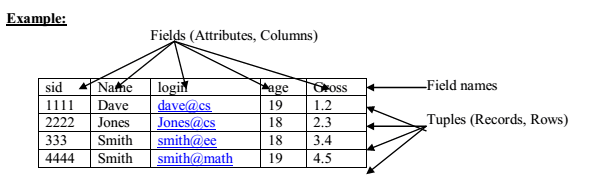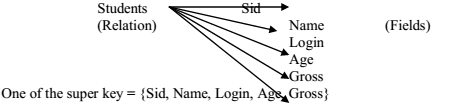# Relational Model A+ A  A-

A database is a collection of 1 or more ‘relations’, where each relation is a table with rows and columns.
This is the primary data model for commercial data processing applications.

The major advantages of the relational model over the older data models are,

1. It is simple and elegant.
2. simple data representation.
3. The ease with which even complex queries can be expressed.

Introduction:
The main construct for representing data in the relational model is a ‘relation’.

A relation consists of

1. Relation Schema.
2. Relation Instance.

Explanation is as below.

1.Relation Schema:
The relation schema describes the column heads for the table.
The schema specifies the relation’s name, the name of each field (column, attribute) and the ‘domain’ of
each field.
A domain is referred to in a relation schema by the domain name and has a set of associated values.

Example:
Student information in a university database to illustrate the parts of a relation schema.Students (Sid: string, name: string, login: string, age: integer, gross: real) This says that the field named ‘sid’ has a domain named ‘string’.
The set of values associated with domain ‘string’ is the set of all character strings

2.Relation Instance:
This is a table specifying the information.
An instance of a relation is a set of ‘tuples’, also called ‘records’, in which each tuple has the same number
of fields as the relation schemas.

A relation instance can be thought of as a table in which each tuple is a row and all rows have the same
number of fields.

The relation instance is also called as ‘relation’.
Each relation is defined to be a set of unique tuples or rows.This example is an instance of the students relation, which consists 4 tuples and 5 fields. No two rows are
identical.

Degree:
The number of fields is called as ‘degree’.
This is also called as ‘arity’.

Cardinality:
The cardinality of a relation instance is the number of tuples in it.

Example:
In the above example, the degree of the relation is 5 and the cardinality is 4.

Relational database:
It is a collection of relations with distinct relation names.

Relational database schema:
It is the collection of schemas for the relations in the database.

Instance:
An instance of a relational database is a collection of relation instances, one per relation schema in the
database schema. Each relation instance must satisfy the domain constraints in its schema.

2.Integrity constraints over relations
An integrity constraint (IC) is a condition that is specified on a database schema and restricts the data can be
stored in an instance of the database.
Various restrictions on data that can be specified on a relational database schema in the form of ‘constraints’.
A DBMS enforces integrity constraints, in that it permits only legal instances to be stored in the database.

Integrity constraints are specified and enforced at different times as below.

1. When the DBA or end user defines a database schema, he or she specifies the ICs that must hold on any instance of this database.
2. When a data base application is run, the DBMS checks for violations and disallows changes to the data that violate the specified ICs.

Legal Instance:
If the database instance satisfies all the integrity constraints specified on the database schema.

The constraints can be classified into 4 types as below.

1. Domain Constraints.
2. Key Constraints.
3. Entity Integrity Constraints.
4. Referential Integrity Constraints.

Explanation is as below.

1.Domain Constraints
Domain constraints are the most elementary form of integrity constraints. They are tested easily by the system
whenever a new data item is entered into the database.

Domain constraints specify the set of possible values that may be associated with an attribute. Such constraints
may also prohibit the use of null values for particular attributes.

The data types associated with domains typically include standard numeric data types for integers

A relation schema specifies the domain of each field or column in the relation instance.
These domain constraints in the schema specify an important condition that each instance of the relation to
satisfy: The values that appear in a column must be drawn from the domain associated with that column.
Thus the domain of a field is essentially the type of that field

2.Key Constraints
1.Explain the concept of Super Key, Candidate Key and Primary Key with examples?(6 Marks, Feb-2004)
A key constraint is a statement that a certain minimal subset of the fields of a relation is a unique identifier for a
tuple.

Example:
The ‘students’ relation and the constraint that no 2 students have tha same student id (sid).

These can be classified into 3 types as below.

1. Candidate Key or Key.
2. Super Key.
3. Primary Key.

Explanation is as below.

a. Candidate Key or Key:

A set of fields that uniquely identifies a tuple according to a key constraint is called as a ‘Candidate Key’ for the
relation. This is also called as a ‘key’.

From the definition of candidate key, we have,

1.Two distinct tuples in a legal instance cannot have identical valuesin all the fields of a key.i.e, in any legal instance, the values in the key fields uniquely identify a tuple in the instance. i.e,the values in the key fields uniquely identify a tuple in the instance.

2. No subset of the set of fields in key is a unique identifier for a tuple,i.e., the set of fields {sid, name} is not a key for Students. A relation schema may have more than key.
Example: In the above Students relation, the ‘sid’ field is a candidate key.
{sid}.

The value of a key attribute can be used to identify uniquely each tuple in the relation.
‘A set of attributes constituting a key’ is a property of the relation schema.
A key is determined from the meaning of attributes.
Every relation is guaranteed to have a key. Since a relation is a set of tuples, the set of all fields is always a super
key.

b. Super Key:
The set of fields that contains a key is called as a ‘super key’.
The set of 1 or more attributes that allows us to identify uniquely an entity in the entity set.
A super key specifies a uniqueness constraint that no 2 distinct tuples can have the same value.
Every relation has at least 1 default super key as the set of all attributes.

Example:c. Primary Key:

This is also a candidate key, whose values are used to identify tuples in the relation.
It is common to designate one of the candidate keys as a primary key of the relation.

The attributes that form the primary key of a relation schema are underlined.
It is used to denote a candidate key that is chosen by the database designer as the
principal means of identifying entities with an entity set.

Example:
‘Sid’ of Students relation.

d. Specifying Key Constraints in SQL-92:
In SQL, we are declaring the set of fields of a table consisting a key by using ‘UNIQUE’ constraint.
This ‘UNIQUE’ constraint specifies that 2 distinct tuples cannot have identical Values.
Candidate keys can be declared as a ‘primary key’ using the constraint ‘PRIMARY KEY’

We can name a constraint by using the syntax as below.
CONSTRAINT constraint_name KEY_NOTATION (key_names);

If the constraint is violated, then the constraint_name is returned and it can be used
to identify the error.

Example:
Express ‘sid’ as a primary key and the combination {name, age} as a key.
CREATE TABLE Students (sid CHAR (20), name CHAR (30), login CHAR(20),age INTEGER, gross REAL, UNIQUE (name, age),` CONSTRAINT sid1 PRIMARY KEY (sid));

3.Entity Integrity Constraints
This states that no primary key value can be null.
The primary key value is used to identify individual tuples in a relation.
Having null values for the primary key implies that we cannot identify some tuples.
NOTE: Key Constraints, Entity Integrity Constraints are specified on individual relations.PRIMARY KEYS comes under this

4.Referential Integrity Constraints
The Referential Integrity Constraint is specified between 2 relations and is used to maintain the consistency among tuples of the 2 relations.Informally, the referential integrity constraint states that ‘a tuple in 1 relation that
refers to another relation must refer to an existing tuple in that relation.

We can diagrammatically display the referential integrity constraints by drawing a
directed arc from each foreign key to the relation it references. The arrowhead may
point to the primary key of the referenced relation.dbms-relational-model